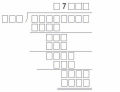用算幾不等式解函數值域...

用算幾不等式解函數值域...附件題目.docx (35.65 KB)

2018-8-17 16:08, 下載次數: 544

TOP

 lyingheart 萊因哈特發短消息 加為好友 當前離線 2# 大 中 小 發表於 2018-8-18 22:54  只看該作者 比較喜歡用幾何 $$\displaystyle f(x)=\frac{\sin x}{\sqrt{4\cos x+4\cos^2 x+4\sin^2 x+1}}$$ $$\displaystyle =\frac{\sin x}{\sqrt{(2\cos x+1)^2+(2\sin x)^2}}$$ 視為 $$x^2+y^2=4$$ 上一點到 $$x$$ 軸距離(有正負)與到點 $$(-1,0)$$ 的距離比值之半。 [ 本帖最後由 lyingheart 於 2018-8-18 22:56 編輯 ] UID1430 帖子90 閱讀權限10 在線時間252 小時 註冊時間2013-4-24 最後登錄2019-8-9  查看詳細資料 TOP
﻿